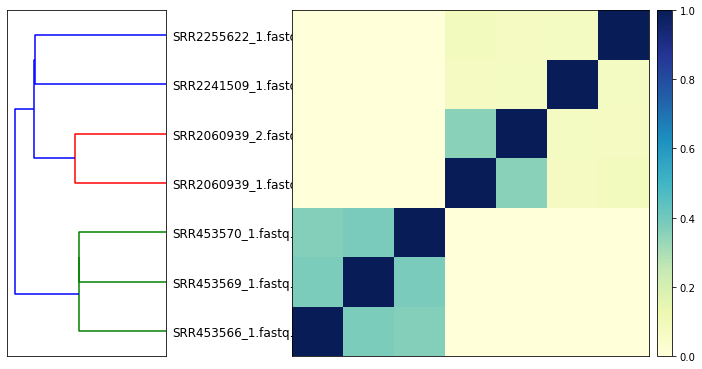# Building plots from sourmash compare output¶

### Running this notebook.¶

You can run this notebook interactively via mybinder; click on this button:A rendered version of this notebook is available at sourmash.readthedocs.io under "Tutorials and notebooks".

You can also get this notebook from the doc/ subdirectory of the sourmash github repository. See binder/environment.yaml for installation dependencies.

### What is this?¶

This is a Jupyter Notebook using Python 3. If you are running this via binder, you can use Shift-ENTER to run cells, and double click on code cells to edit them.

Contact: C. Titus Brown, [email protected] Please file issues on GitHub if you have any questions or comments!

## Running sourmash compare and generating figures in Python¶

First, we need to generate a similarity matrix with compare. (If you want to generate this programmatically, it's just a numpy matrix.)

In :
!sourmash compare ../tests/test-data/demo/*.sig -o compare-demo

== This is sourmash version 3.3.2.dev9+g462bc387. ==
== Please cite Brown and Irber (2016), doi:10.21105/joss.00027. ==

loaded 1 sigs from '../tests/test-data/demo/SRR2060939_1.sig'g'
loaded 1 sigs from '../tests/test-data/demo/SRR2060939_2.sig'g'
loaded 1 sigs from '../tests/test-data/demo/SRR2241509_1.sig'g'
loaded 1 sigs from '../tests/test-data/demo/SRR2255622_1.sig'g'
loaded 1 sigs from '../tests/test-data/demo/SRR453566_1.sig'g'
loaded 1 sigs from '../tests/test-data/demo/SRR453569_1.sig'g'
loaded 1 sigs from '../tests/test-data/demo/SRR453570_1.sig'g'
loaded 7 signatures total.

0-SRR2060939_1.fa...	[1.    0.356 0.078 0.086 0.    0.    0.   ]
1-SRR2060939_2.fa...	[0.356 1.    0.072 0.078 0.    0.    0.   ]
2-SRR2241509_1.fa...	[0.078 0.072 1.    0.074 0.    0.    0.   ]
3-SRR2255622_1.fa...	[0.086 0.078 0.074 1.    0.    0.    0.   ]
4-SRR453566_1.fas...	[0.    0.    0.    0.    1.    0.382 0.364]
5-SRR453569_1.fas...	[0.    0.    0.    0.    0.382 1.    0.386]
6-SRR453570_1.fas...	[0.    0.    0.    0.    0.364 0.386 1.   ]
min similarity in matrix: 0.000
saving labels to: compare-demo.labels.txt
saving comparison matrix to: compare-demo

In :
%pylab inline
# import the fig module from sourmash:
from sourmash import fig

Populating the interactive namespace from numpy and matplotlib


The sourmash.fig module contains code to load the similarity matrix and associated labels:

In :
matrix, labels = fig.load_matrix_and_labels('compare-demo')


Here, matrix is a numpy matrix and labels is a list of labels (by default, filenames).

In :
print('matrix:\n', matrix)
print('labels:', labels)

matrix:
[[1.    0.356 0.078 0.086 0.    0.    0.   ]
[0.356 1.    0.072 0.078 0.    0.    0.   ]
[0.078 0.072 1.    0.074 0.    0.    0.   ]
[0.086 0.078 0.074 1.    0.    0.    0.   ]
[0.    0.    0.    0.    1.    0.382 0.364]
[0.    0.    0.    0.    0.382 1.    0.386]
[0.    0.    0.    0.    0.364 0.386 1.   ]]
labels: ['SRR2060939_1.fastq.gz', 'SRR2060939_2.fastq.gz', 'SRR2241509_1.fastq.gz', 'SRR2255622_1.fastq.gz', 'SRR453566_1.fastq.gz', 'SRR453569_1.fastq.gz', 'SRR453570_1.fastq.gz']


The plot_composite_matrix function returns a generated plot, along with the labels and matrix as re-ordered by the clustering:

In :
f, reordered_labels, reordered_matrix = fig.plot_composite_matrix(matrix, labels)In :
print('reordered matrix:\n', reordered_matrix)
print('reordered labels:', reordered_labels)

reordered matrix:
[[1.    0.382 0.364 0.    0.    0.    0.   ]
[0.382 1.    0.386 0.    0.    0.    0.   ]
[0.364 0.386 1.    0.    0.    0.    0.   ]
[0.    0.    0.    1.    0.356 0.078 0.086]
[0.    0.    0.    0.356 1.    0.072 0.078]
[0.    0.    0.    0.078 0.072 1.    0.074]
[0.    0.    0.    0.086 0.078 0.074 1.   ]]
reordered labels: ['SRR2255622_1.fastq.gz', 'SRR2241509_1.fastq.gz', 'SRR2060939_2.fastq.gz', 'SRR2060939_1.fastq.gz', 'SRR453570_1.fastq.gz', 'SRR453569_1.fastq.gz', 'SRR453566_1.fastq.gz']


## Customizing plots¶

If you want to customize the plots, please see the code for plot_composite_matrix in sourmash/fig.py, which is reproduced below; you can modify the code in place to (for example) use custom dendrogram colors.

In :
import scipy.cluster.hierarchy as sch

def plot_composite_matrix(D, labeltext, show_labels=True, show_indices=True,
vmax=1.0, vmin=0.0, force=False):
"""Build a composite plot showing dendrogram + distance matrix/heatmap.
Returns a matplotlib figure."""
if D.max() > 1.0 or D.min() < 0.0:
error('This matrix doesn\'t look like a distance matrix - min value {}, max value {}', D.min(), D.max())
if not force:
raise ValueError("not a distance matrix")
else:
notify('force is set; scaling to [0, 1]')
D -= D.min()
D /= D.max()

if show_labels:
show_indices = True

fig = pylab.figure(figsize=(11, 8))
ax1 = fig.add_axes([0.09, 0.1, 0.2, 0.6])

# plot dendrogram
Y = sch.linkage(D, method='single')  # centroid

dendrolabels = labeltext
if not show_labels:
dendrolabels = [str(i) for i in range(len(labeltext))]

Z1 = sch.dendrogram(Y, orientation='left', labels=dendrolabels,
no_labels=not show_indices)
ax1.set_xticks([])

xstart = 0.45
width = 0.45
if not show_labels:
xstart = 0.315
scale_xstart = xstart + width + 0.01

# plot matrix
axmatrix = fig.add_axes([xstart, 0.1, width, 0.6])

# (this reorders D by the clustering in Z1)
idx1 = Z1['leaves']
D = D[idx1, :]
D = D[:, idx1]

# show matrix
im = axmatrix.matshow(D, aspect='auto', origin='lower',
cmap=pylab.cm.YlGnBu, vmin=vmin, vmax=vmax)
axmatrix.set_xticks([])
axmatrix.set_yticks([])

# Plot colorbar.
axcolor = fig.add_axes([scale_xstart, 0.1, 0.02, 0.6])
pylab.colorbar(im, cax=axcolor)

return fig

In :
_ = plot_composite_matrix(matrix, labels)